Question

# Question 4 A control system is shown in Figure 3, where Ge(s) is the controller, R(s)...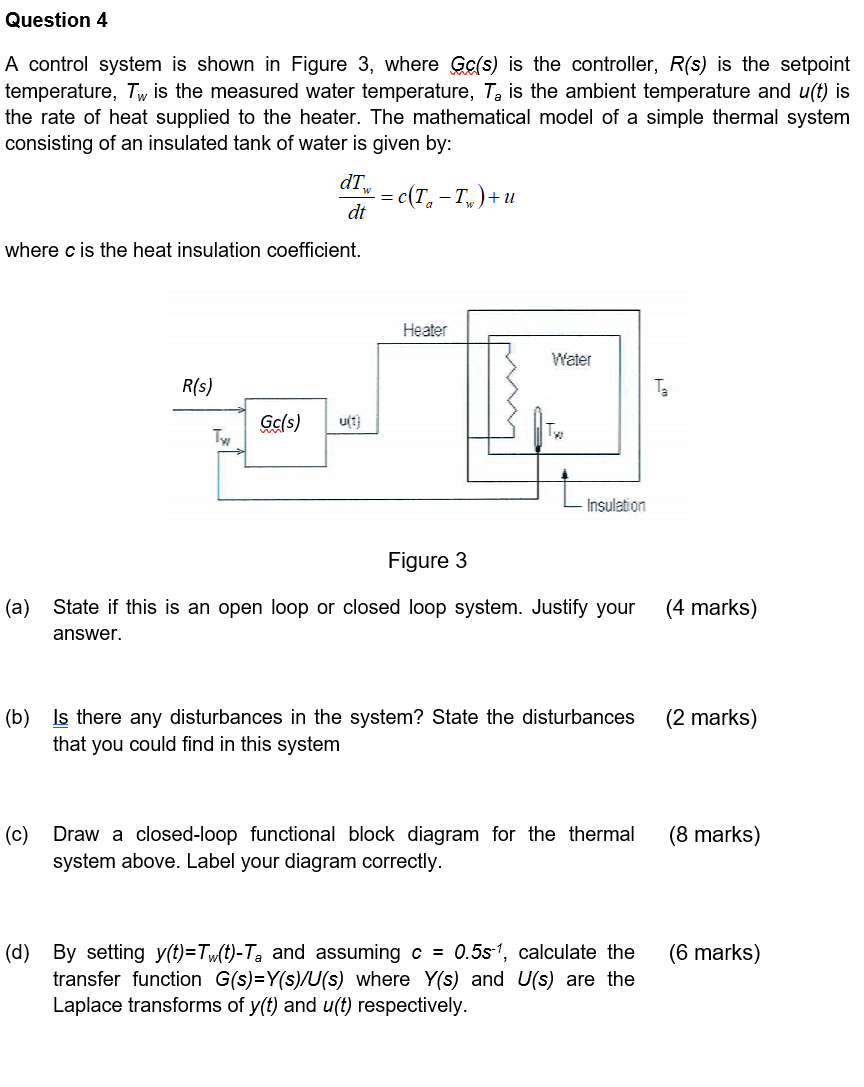Question 4 A control system is shown in Figure 3, where Ge(s) is the controller, R(s) is the setpoint temperature, Tw is the measured water temperature, Ta is the ambient temperature and u(t) is the rate of heat supplied to the heater. The mathematical model of a simple thermal system consisting of an insulated tank of water is given by: dTW = c(1, - Iw) +u dt where c is the heat insulation coefficient. Heater Water R(s) Ta Gc/s) u(1) TW Insulation Figure 3 (a) State if this is an open loop or closed loop system. Justify your answer. (4 marks) (2 marks) (b) Is there any disturbances in the system? State the disturbances that you could find in this system (8 marks) (c) Draw a closed-loop functional block diagram for the thermal system above. Label your diagram correctly. (6 marks) (d) By setting y(t)=Tw(t)-Ta and assuming c = 0.55-1, calculate the transfer function G(s)=Y(s)/U(s) where y(s) and U(s) are the Laplace transforms of y(t) and u(t) respectively.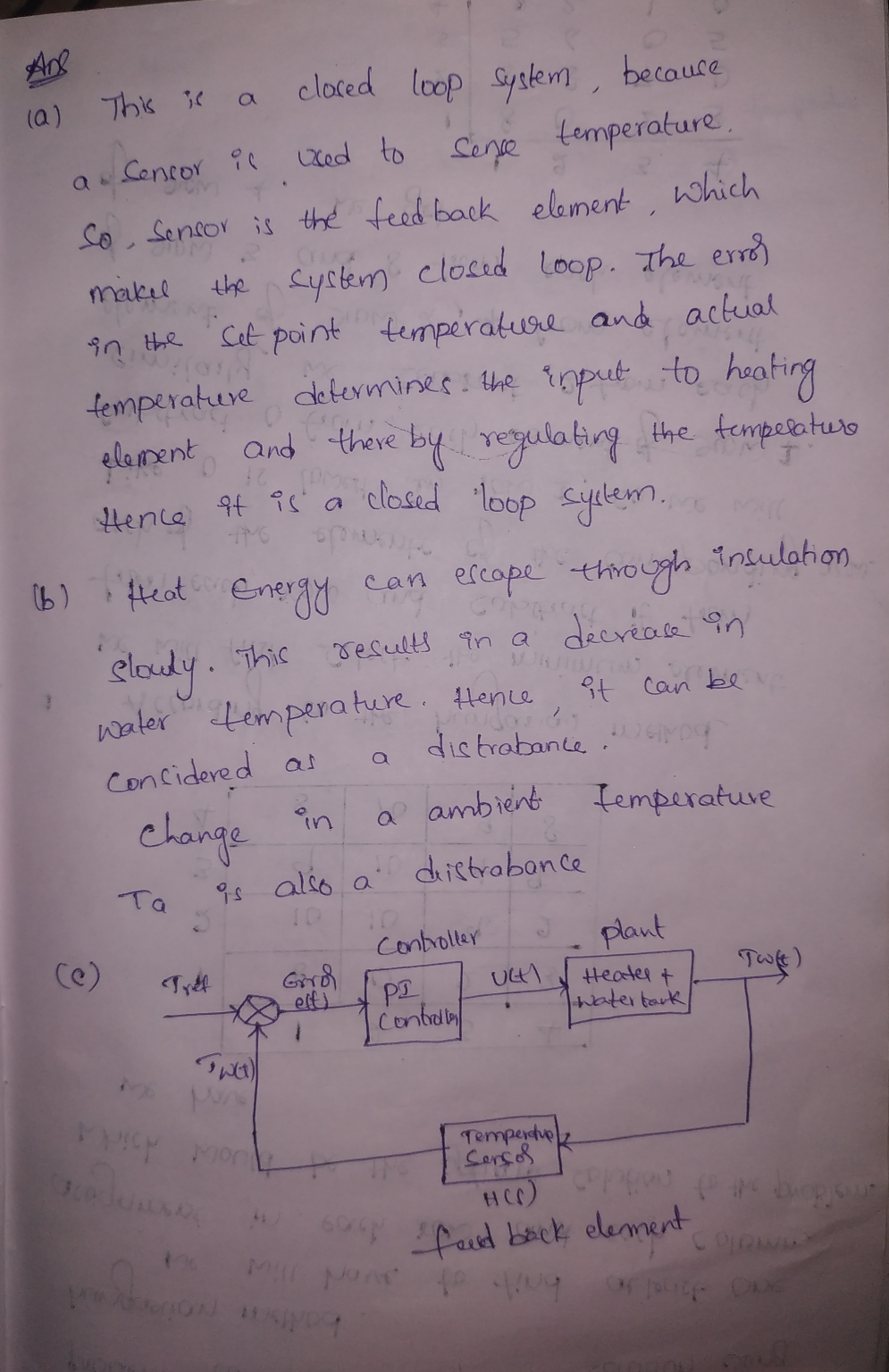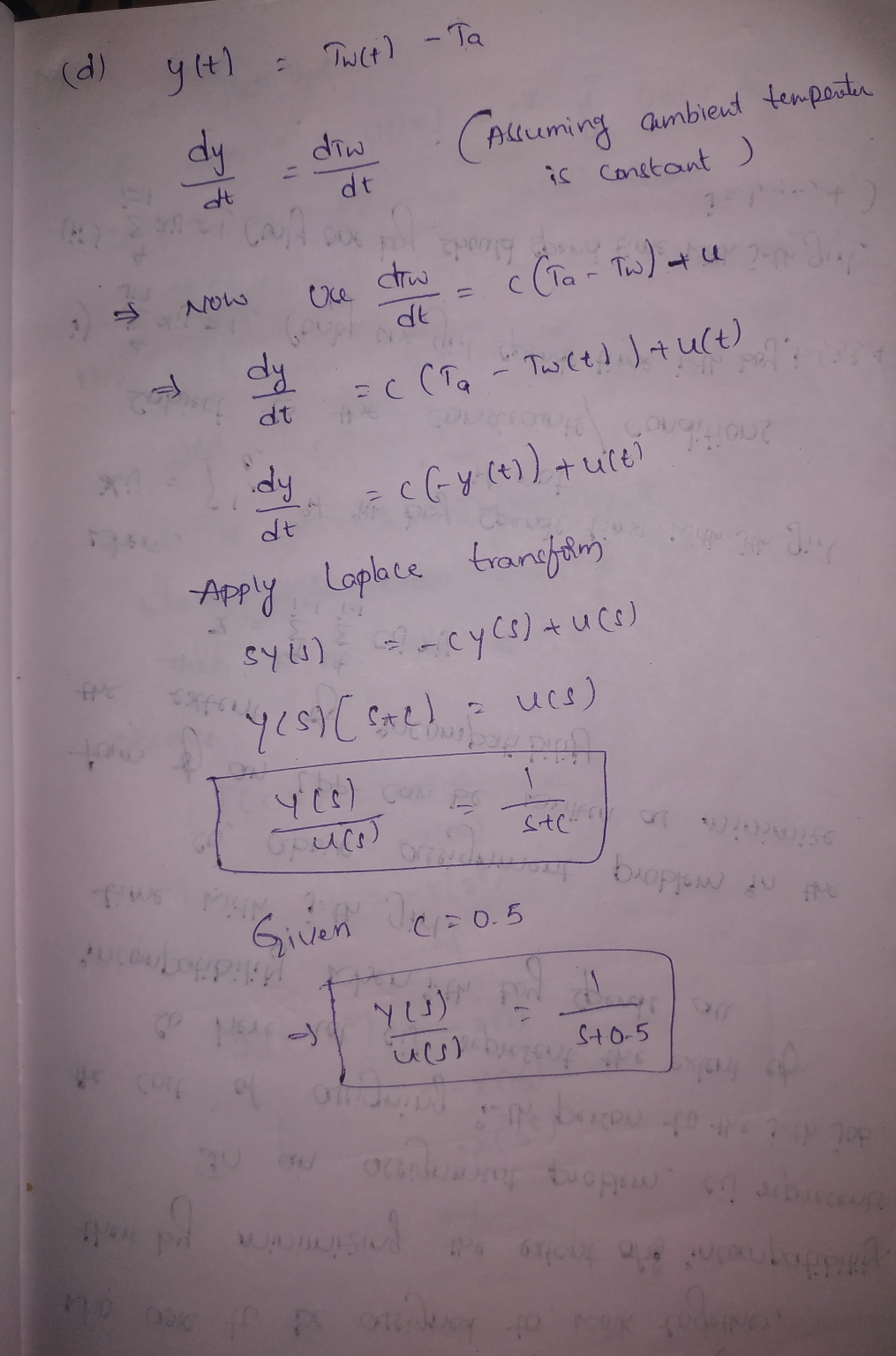#### Earn Coins

Coins can be redeemed for fabulous gifts.

Similar Homework Help Questions
• ### Question 3 (10 +10+10+15 45 marks) E(s) C(s) R(s) Figure 3: Unity feedback control system for Question 3 For the unity...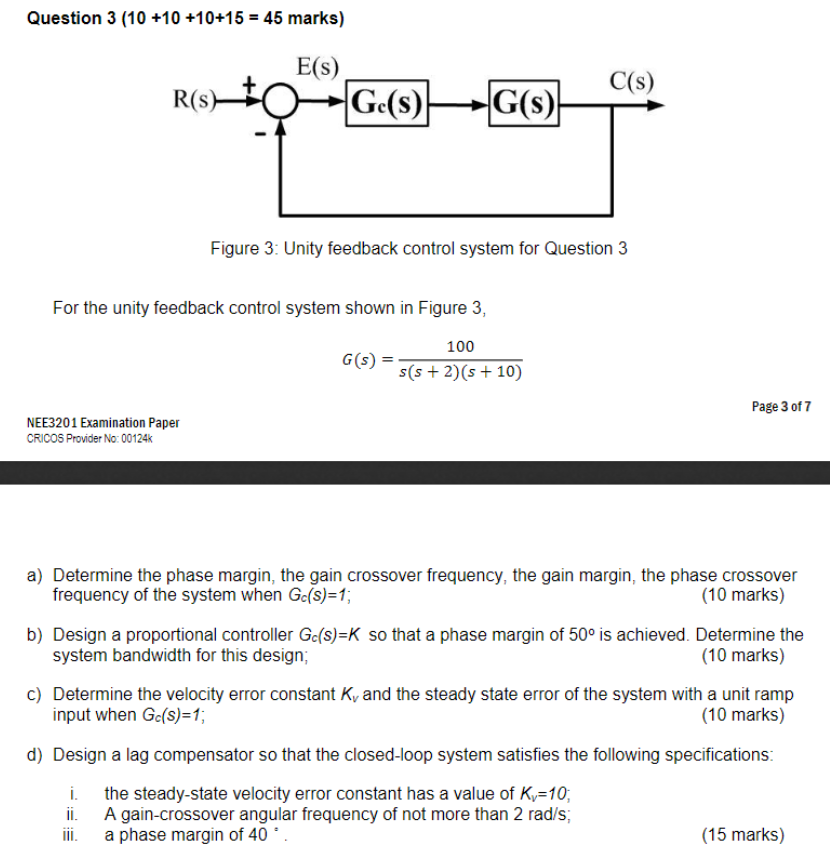Question 3 (10 +10+10+15 45 marks) E(s) C(s) R(s) Figure 3: Unity feedback control system for Question 3 For the unity feedback control system shown in Figure 3, 100 G(S) (s+2)(+10) Page 3 of 7 NEE3201 Examination Paper CRICOS Provider No: 00124k a) Determine the phase margin, the gain crossover frequency, the gain margin, the phase crossover frequency of the system when Gc(s)-1, 10 marks) b) Design a proportional controller Gc(s)-K so that a phase margin of 50° is achieved....

• ### For the following thermal heating system shown below: Controller Process E (8) Ris) Gs) G(s) Y8)...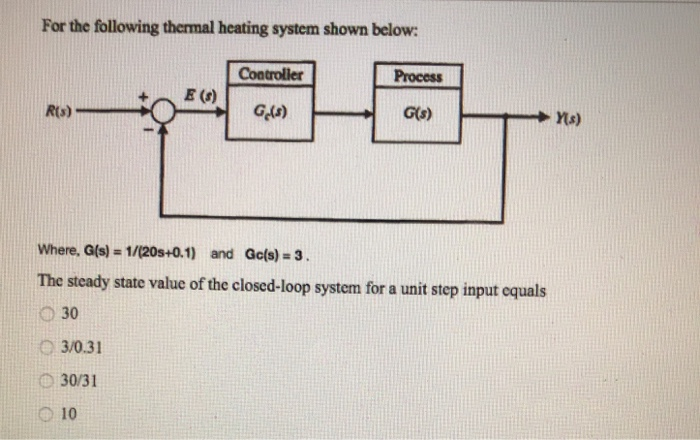For the following thermal heating system shown below: Controller Process E (8) Ris) Gs) G(s) Y8) Where, G(s) = 1/(20s+0.1) and Gc(s) = 3. The steady state value of the closed-loop system for a unit step input equals 30 3/0.31 30/31 10

• ### Question 4 (a) A feedback control system with a proportional controller is shown in Figure Q4...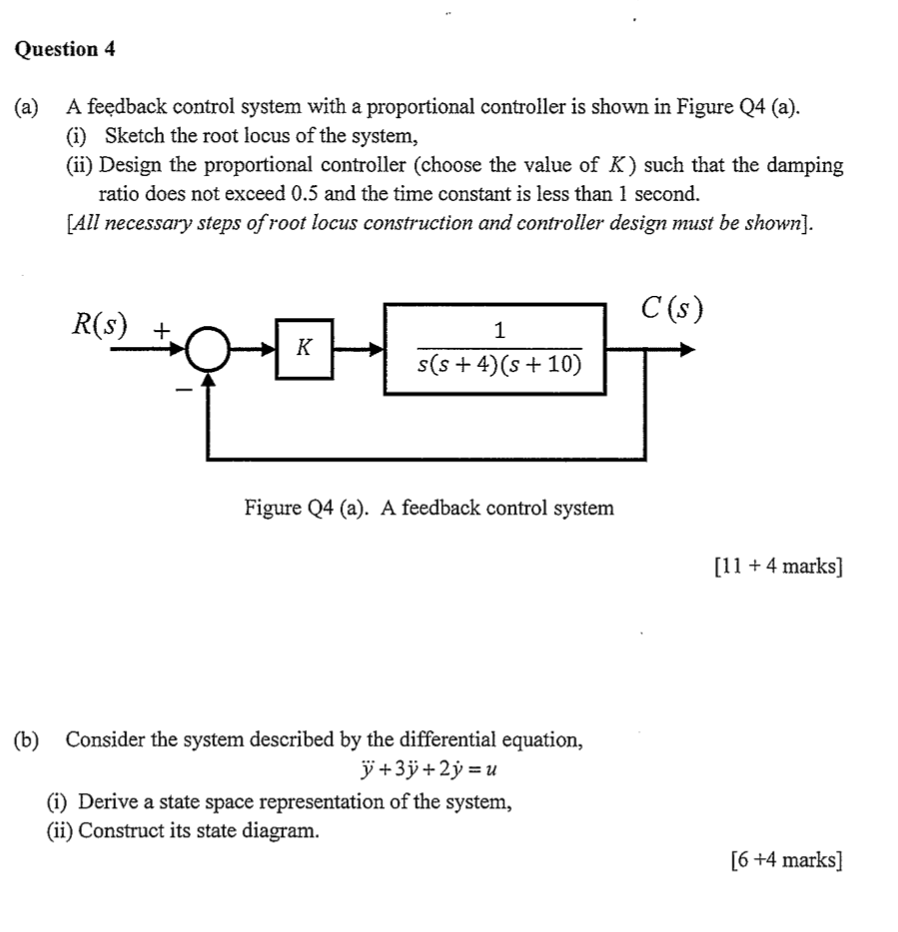Question 4 (a) A feedback control system with a proportional controller is shown in Figure Q4 (a). (i) Sketch the root locus of the system, (ii) Design the proportional controller (choose the value of K) such that the damping ratio does not exceed 0.5 and the time constant is less than 1 second. [All necessary steps of root locus construction and controller design must be shown). C(s) R(S) + s(s+4)(s + 10) Figure Q4 (a). A feedback control system [11...

• ### Wis) R(s u(s) 14 Gl(s) H(s) Given a system as in the diagram above, where K is an adjustable pa...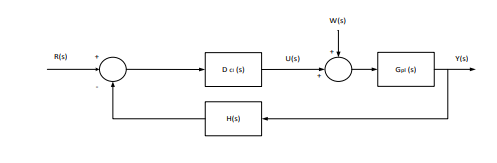Wis) R(s u(s) 14 Gl(s) H(s) Given a system as in the diagram above, where K is an adjustable parameter pl(s) Dal(sKp+ g) Assuming W-0, find the transfer function Y(s)/R(s) h) Assuming R-0, find the transfer function Y(s)/W(s) i) What is the type of the system (with respect to steady-state error)? j) What is the steady-state error when rt)u(t) (unit-step) and w(t)-0 k) What is the s.s. error when r(t) t u(t) and w(t)-0 ) Assume r(t)-0, what is the...

• ### for what ?? Question/12_ The room shown in the figure has a heater with heat flow...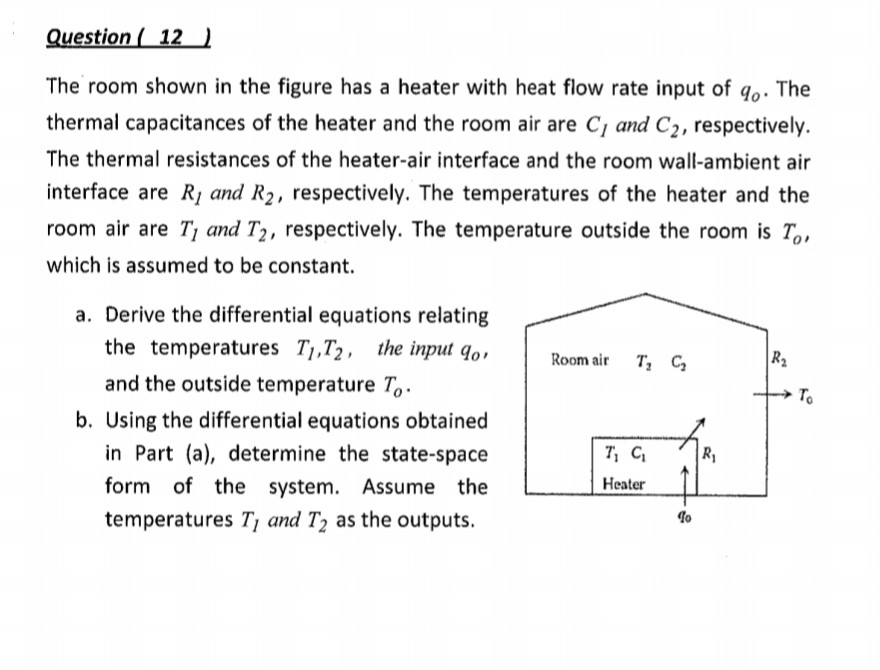for what ?? Question/12_ The room shown in the figure has a heater with heat flow rate input of go. The thermal capacitances of the heater and the room air are C, and C2, respectively. The thermal resistances of the heater-air interface and the room wall-ambient air interface are R, and R2, respectively. The temperatures of the heater and the room air are T, and T2, respectively. The temperature outside the room is T. which is assumed to be constant....

• ### Q.4 A position control system is shown in Figure Q4. Assume that K(s) = K, the plant 50 s(0.2s +1) transfer function is given by G(s) s02s y(t) r(t) Figure Q4: Feedback control system. (a) Design a l...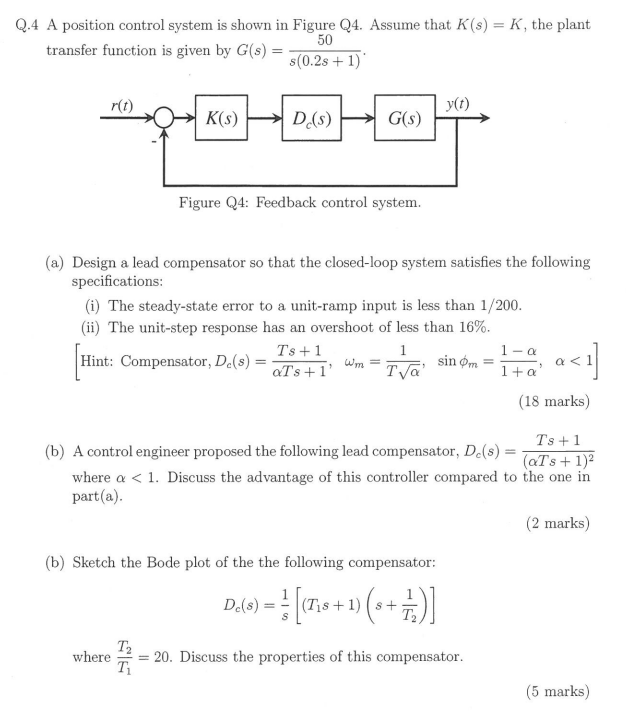Q.4 A position control system is shown in Figure Q4. Assume that K(s) = K, the plant 50 s(0.2s +1) transfer function is given by G(s) s02s y(t) r(t) Figure Q4: Feedback control system. (a) Design a lead compensator so that the closed-loop system satisfies the following specifications (i) The steady-state error to a unit-ramp input is less than 1/200 (ii) The unit-step response has an overshoot of less than 16% Ts +1 Hint: Compensator, Dc(s)=aTs+ 1, wm-T (18 marks)...

• ### Could someone help me with this question please as I am kinda lost as in how to do these type of questions please explain each step a) Draw a diagram of a solar hot water system and discuss the funct...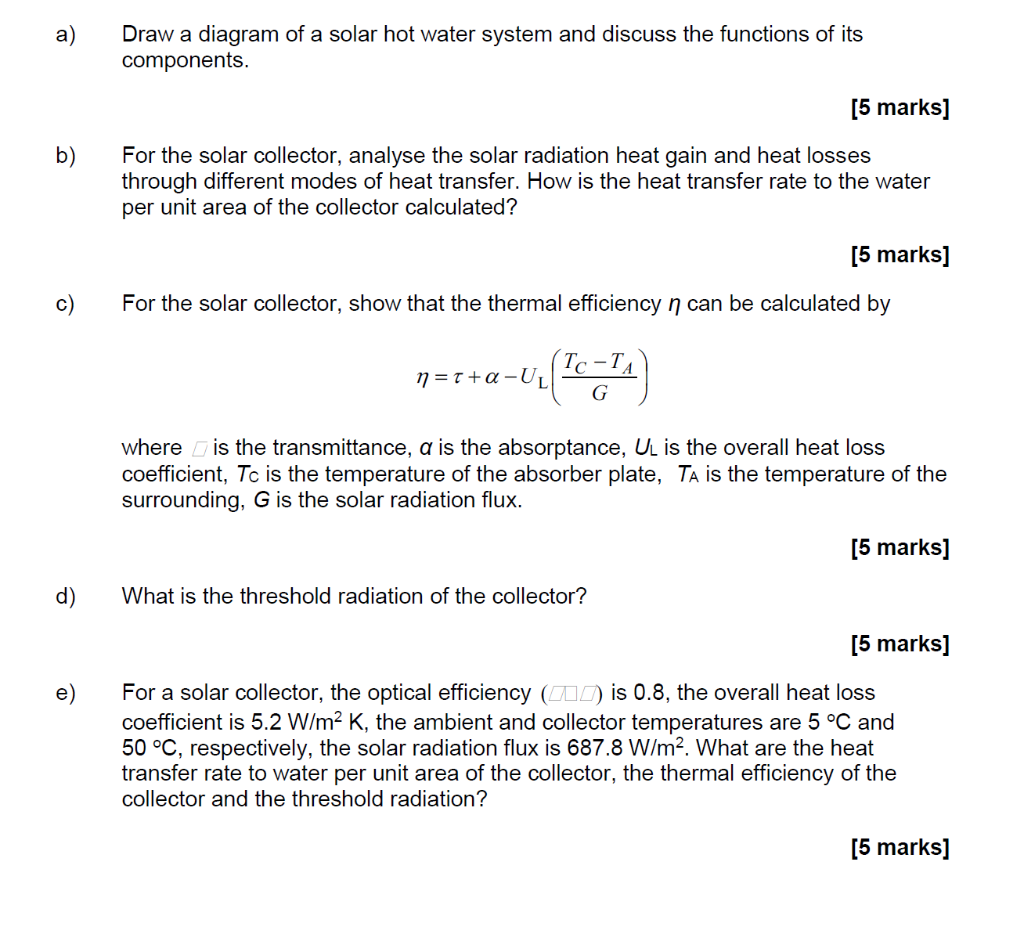Could someone help me with this question please as I am kinda lost as in how to do these type of questions please explain each step a) Draw a diagram of a solar hot water system and discuss the functions of its [5 marks For the solar collector, analyse the solar radiation heat gain and heat losses through different modes of heat transfer. How is the heat transfer rate to the water per unit area of the collector calculated? b)...

• ### 1. [25%] Consider the closed-loop system shown where it is desired to stabilize the system with...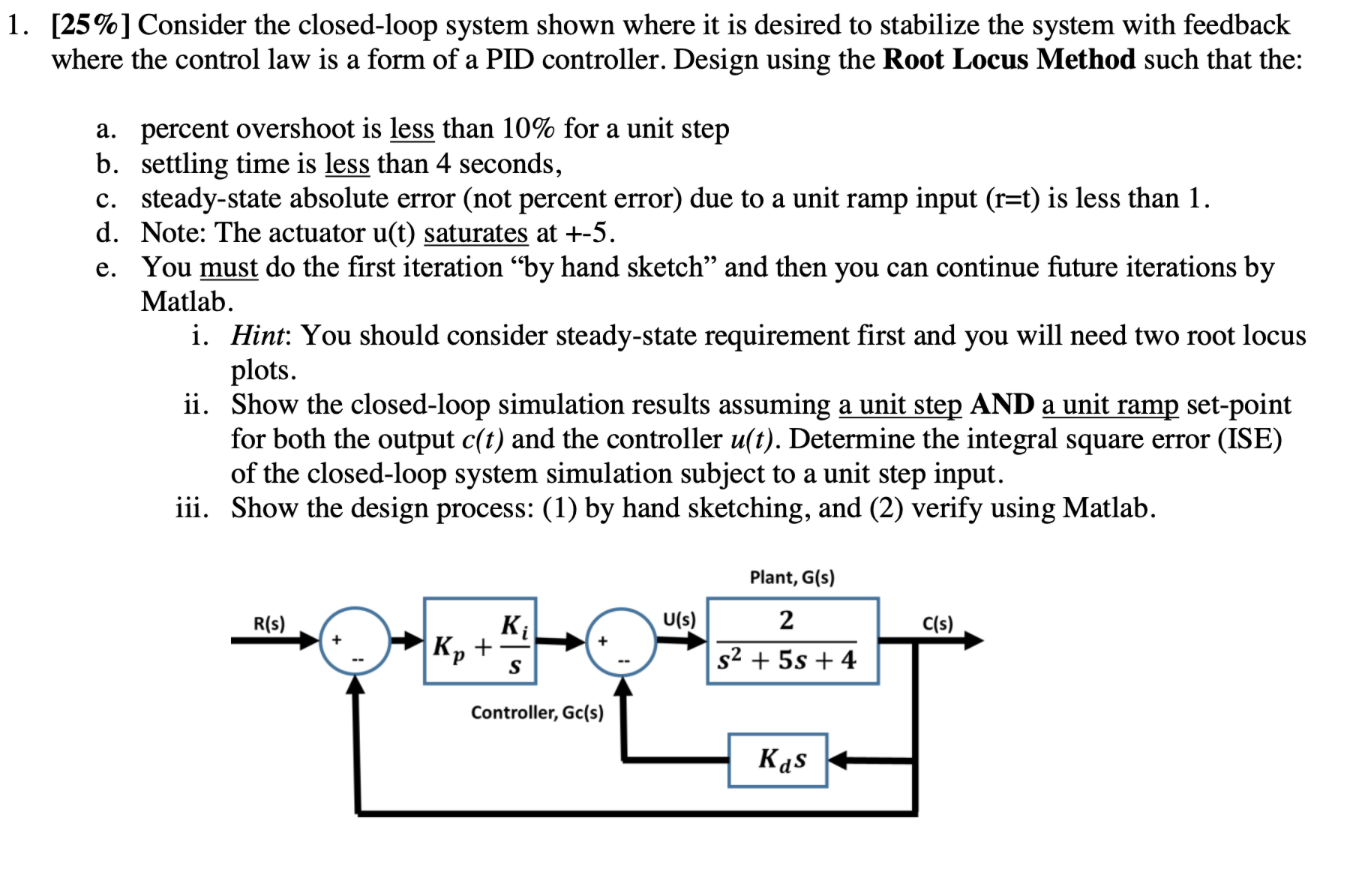1. [25%] Consider the closed-loop system shown where it is desired to stabilize the system with feedback where the control law is a form of a PID controller. Design using the Root Locus Method such that the: a. percent overshoot is less than 10% for a unit step b. settling time is less than 4 seconds, c. steady-state absolute error (not percent error) due to a unit ramp input (r=t) is less than 1. d. Note: The actuator u(t) saturates...

• ### C(8) for the system shown in Figure 1. R(S Find the equivalent transfer function, Geg (s)...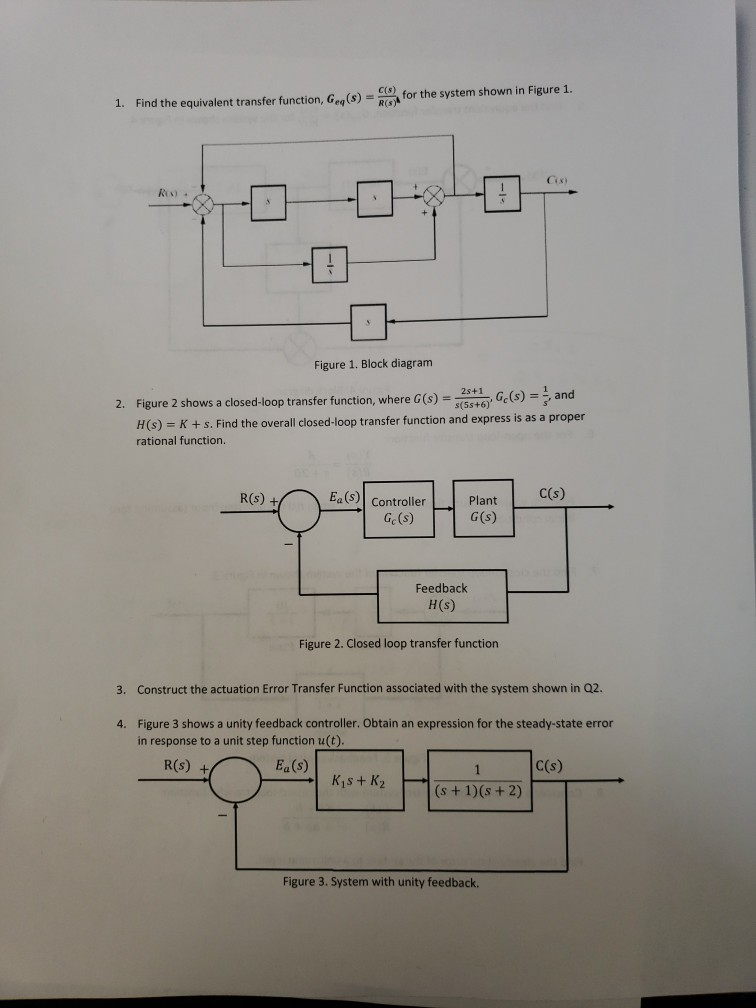C(8) for the system shown in Figure 1. R(S Find the equivalent transfer function, Geg (s) 1 Cix) Figure 1. Block diagram 2s+1 s(5s+6Ge(s) = and Figure 2 shows a closed-loop transfer function, where G(s) 2. proper H(s) K+s. Find the overall closed-loop transfer function and express is as rational function. C(s) Ea (s) Controller R(s) +/ Plant G(s) Ge (s) Feedback H(s) Figure 2. Closed loop transfer function Construct the actuation Error Transfer Function associated with the system shown...

• ### 1. A feedback control system is shown in the figure below. Suppose that our design objective...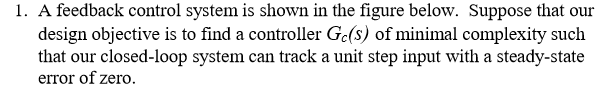1. A feedback control system is shown in the figure below. Suppose that our design objective is to find a controller Gc(S) of minimal complexity such that our closed-loop system can track a unit step input with a steady-state error of zero. (b) Now consider a more complex controller Gc(S) = [ Ko + K//s] where Ko = 2 and Ki = 20. (This is a proportional + integral (PI) controller). Plot the unit step response, and determine the steady-state...